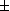# Function draw --- Introduction ---

Function draw is an exercise on the graphic recognition of functions of one real variable. The server will give you the graph of a function f(x), whose expression will be hidden. Then you are asked to draw the graph of another function such as f(-x), 2f(x), f(x-1), etc (see the menu below), with the mouse. You will have a score according to the precision of your drawing.
You can choose the software used

The exercise can be configured by the following parameters.

• Type of the function:f(x) f(x1), f(x)1 f(x1)f(x)1 f(2x),f(x/2),2f(x),f(x)/2|f(x)|,f(|x|) f(x2),f2(x) f(max/min(1,x)) max/min(1,f(x)) max/min(x,f(x))
• Number of drawings in one session: , , , , , , , (The score is attributed only at the end of a session.)
• Severity level: , , , , , , , , ,

Other exercises on: java draws   Functions

In order to access WIMS services, you need a browser supporting forms. In order to test the browser you are using, please type the word wims here: and press Enter''.